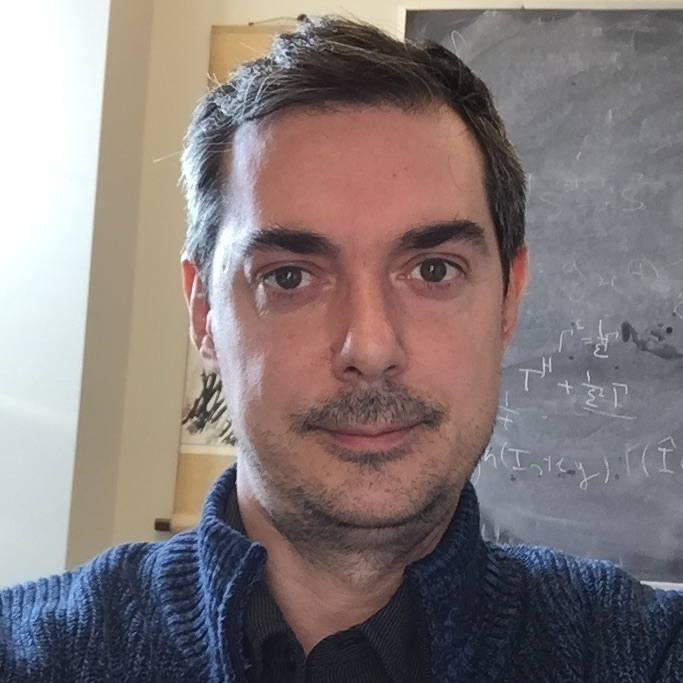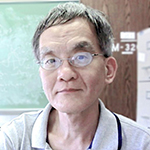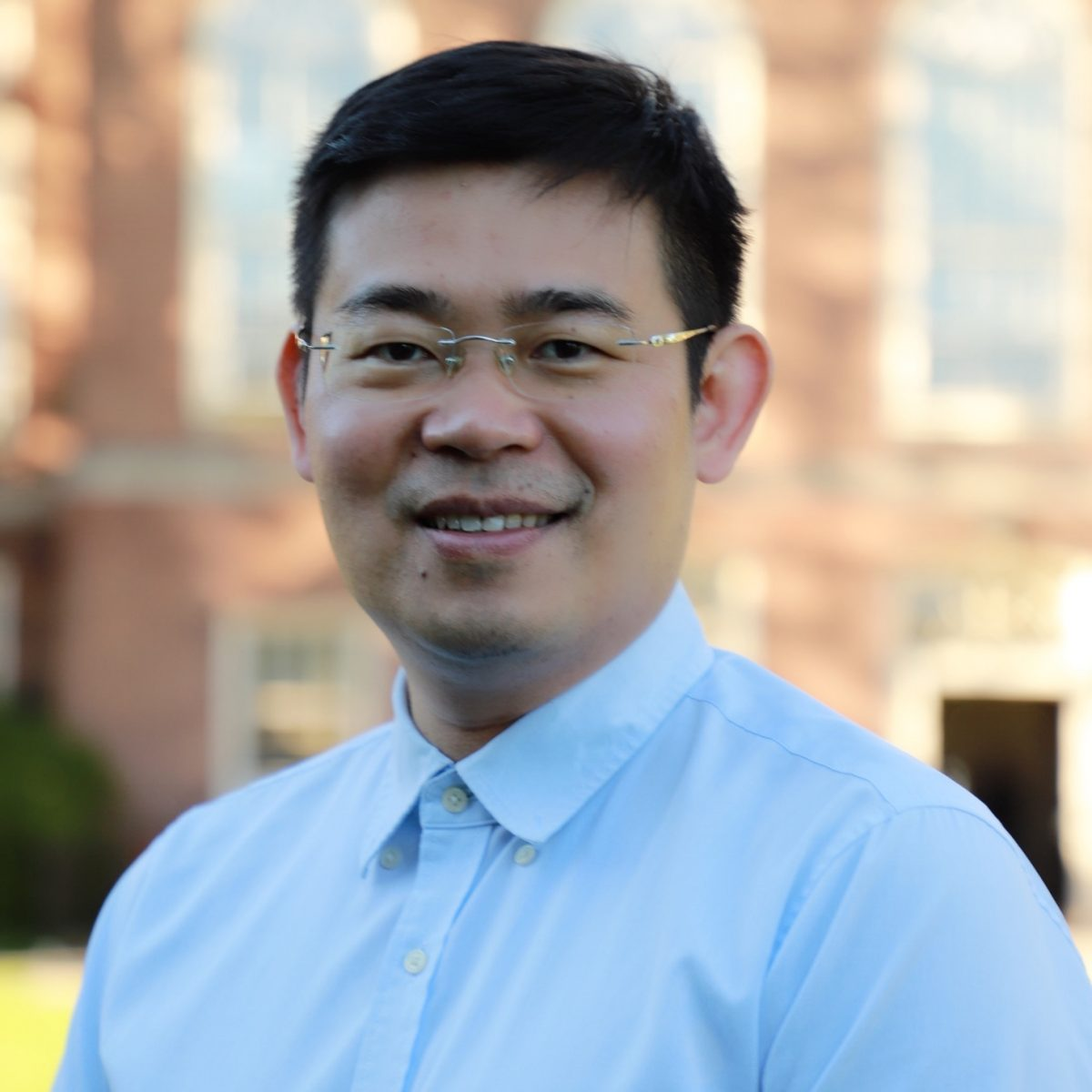# Partial Differential Equations

## Research Activity

Partial Differential Equations show up in almost all fields of exact sciences. Within this broad scope, research at UConn’s math department focuses mainly on the following topics:

• Linear partial differential equations and Brownian motion.
• Nonlinear Analysis: the Mountain Pass Theorem.
• The Gierer Meinhardt model.
• Bose Einstein condensates.
• Calculus of variations.
• Fully nonlinear partial differential equations and its applications to differential geometry and algebraic geometry.
• Geometric partial differential equations that arises naturally from mathematical general relativity.
• Elliptic and parabolic equations from materials science and continuum mechanics.

## Members#### Fabrice Baudoin#### Fabiana Cardetti#### Yung Choi#### Lan-Hsuan Huang#### Dmitriy Leykekhman#### Guozhen Lu#### Damin Wu#### Ling Xiao